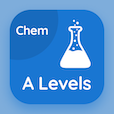Cambridge Online Courses (GCE)

A Level Chemistry MCQs

A Level Chemistry MCQ PDF - Topics

# Introduction to Alcohols MCQ Quiz Online

Learn Introduction to Alcohols Multiple Choice Questions (MCQ), Introduction to Alcohols quiz answers PDF to study a level chemistry online course for a level chemistry classes. Alcohols and Esters Multiple Choice Questions and Answers (MCQs), Introduction to Alcohols quiz questions for online college courses. "Introduction to Alcohols MCQ" PDF Book: alcohols and esters test prep for best two year degrees.

"The molecules of alcohol have" MCQ PDF: introduction to alcohols with choices hydrogen bonding, dipole forces, van der waals's forces, and covalent bonding for online college courses. Study introduction to alcohols quiz questions for merit scholarship test and certificate programs for GRE subject tests.

## MCQs on Introduction to Alcohols Quiz

MCQ: The molecules of alcohol have

hydrogen bonding
dipole forces
Van der Waals's forces
covalent bonding

MCQ: The general formula for alcohols is

CnH2n
CnH2n+1
CnH
CH3

MCQ: The organic molecules containing hydroxyl group are called

esters
alcohols
aldehydes
ethane

MCQ: 2 methyl-2 propan-2-ol is an example of

primary alcohol
secondary alcohol
tertiary alcohol
quaternary alcohol

MCQ: Organic molecules with similar relative molecular mass have alcohols (CnH2n+1OH) bearing high

boiling point
polarity
volatility
Sublimation point

### More Topics from A Level Chemistry Course